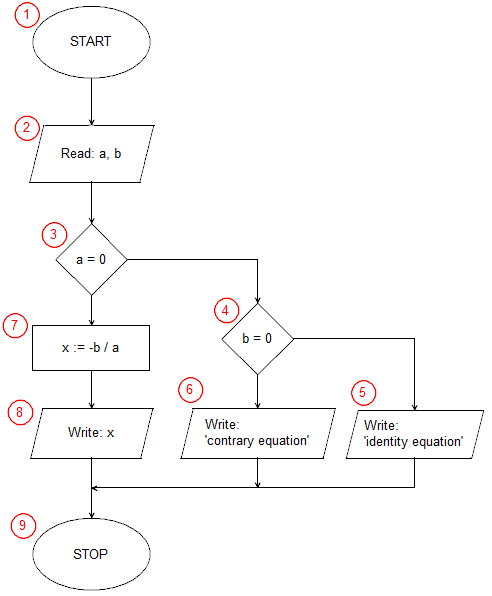# Algorithm of solving the linear equation ax + b = 0

Our task is to find a solution to the linear equation. The general form of the linear equation can be written as follows:

ax + b = 0

where a and b are known real numbers, called equation coefficients. We are looking for such a value, or such values, for the variable x, for which this equation will be fulfilled - the left side of equality will be equal to the right one. The first transformation we make by solving the equation is transferring with the changed sign of the coefficient b (without the unknown) to the right side of the equation (more precisely: we add -b to both sides of the equation). We get:

x = -b

Another transforming is to divide both sides of the equation by the factor a, so that on the left side of the equation only x remains. Before we do, we remind ourselves that the coefficient a is a fixed, known real number, in particular it may be equal to 0, and for 0 to divide we can not. Before doing the division, we must check whether a is different from 0. If so, we will get a solution to our equation:

x = -b / a (a ≠ 0)

The algorithm must still take into account the case of a = 0. In this case, our equation looks as follows:

0 · x = b

Of course 0 · x for each value of x is always equal to 0, i.e. we get:

0 = -b

The left side of the above equality equals the right side only when b=0. Thus, when the coefficient of a linear equation is equal to 0 we deal with two possible situations: b=0 - the equation is fulfilled for each x value (this equation is called the identity equation) and b≠0 - the equation is never met (such equation we call the contradictory equation).

Let's summarize our knowledge about linear equations:

1. When a ≠ 0 the equation has one solution x = -b / a.
2. When a = 0 we have two options:
• b = 0 - identity equation, the solution is any x number belonging to the set of real numbers (infinitely many solutions),
• b ≠ 0 - b ≠ 0 - contradictory equation (no solutions).

Finally, the algorithm for solving the linear equation ax + b = 0, including all possible cases, will look like this:Implementation of the algorithm in: Pascal, C++, Java, Python, JavaScript

Description of the algorithm:

1. Start - our algorithm starts here.
2. We load input data - coefficients a and b of the equation.
3. We check if the coefficient of the equation is equal to 0.
4. If a = 0 we check if the coefficient b of equation equals 0.
5. b = 0 - we print information that the equation is an identity equation.
6. b ≠ 0 - we print information that the equation is a contradictory equation.
7. a ≠ 0 - we calculate the solution of the equation x = -b / a.
8. a ≠ 0 - we print the result (the previously calculated value of x).
9. Stop - the end of the algorithm common to all roads